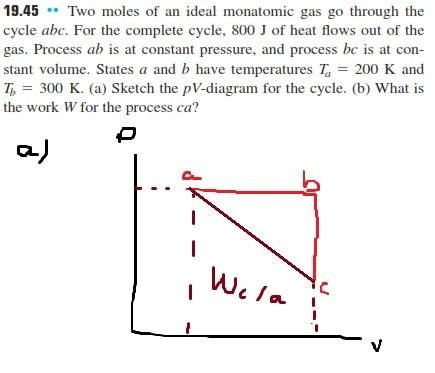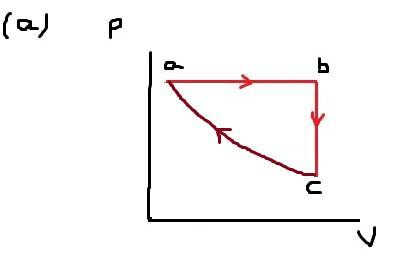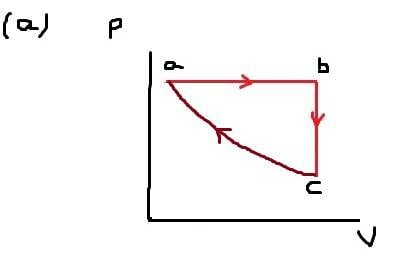What is the work done?

Homework Statementno

The Attempt at a Solution

[/B]
I assumed the path from c to a to be a straight line and I'm unable to find the work as the area in the image above. (sorry my poor english).

The book's answer is -2460 J. The answer I found is ≅ -1112 J.

My calculations:

Va / Ta = Vb / Tb
Vb = 3 Va / 2
P = n R Ta / Va
ΔUfrom a to c = -800J - P Va / 2 = n (3/2) R (Tc - 200K)
Tc = 101.26K

Here is the problem. The area in the graph above should be the work done, and it would be equal to (Pa + Pc) (Va - Vb) / 2
I've used Vb because Vc is equal to it. But solving this expression I cant find the answer of the book.

Last edited:

Simon Bridge
Homework Helper
Its hard to see bc you took the long way around but it looks like your geometry is a bit out or you subtracted someplace you should have added.

Did you try ##W_{cb} = (800J)-W_{ab}## (do you see why that is?)

Ok. I will show you my work in a while.
Did you try Wcb=(800J)−WabW_{cb} = (800J)-W_{ab} (do you see why that is?)
but since process bc is at constant volume, the work there is zero

Ok. I will show you my work in a while.

but since process bc is at constant volume, the work there is zero

I indeed didn't find the answer yet exactly, but process bc is at different pressure, and it should be at different temperature, so there must be some work also

Simon Bridge
Homework Helper
kent said:
...but since process bc is at constant volume, the work there is zero
... yes. Typo ... let me try that again:
##W_{ca} = (800\text{J}) - W_{ab}##

Aside:
Said said:
process bc is at different pressure, and it should be at different temperature, so there must be some work also
In thermodynamics, "work" refers to mechanical work of the whole system: work from moving stuff around.
The system moves stuff around by pushing or pulling on it ... which, since it's closed, it can only do by changing volume.

It's like pushing on a brick wall: since the wall does not move, the mechanical work you do on it is zero even though you get tired. You are using up energy to do zero work on the wall. Where does that energy go? Well, your muscles get warm...

ehild
Homework Helper

The Attempt at a Solution

[/B]
I assumed the path from c to a to be a straight line and I'm unable to find the work as the area in the image above.

You can not assume that the path from c to a was a straight line. There was work done on the gas, and heat transfer at the same time.
You only know that 800 J heat was removed from the gas during the whole cycle. It was a cycle, so the internal energy returned to its initial value. So what was the work done on the gas during the whole process?

•Simon Bridge
Oh okay Simon Bridge. Thank you ehild for remembering that it was a cycle, and in this case the net change in the internal energy is zero. But yet, I've found a answer greater than -2460 J.
P = n R Ta / Va
Vb = 3 Va / 2
Wfrom a to b = P (Vb - Va) = (n R Ta / Va) 0.5 Va = 1662.8 J
Wfrom a to b + Wfrom c to a = Q = -800 J
Wfrom c to a = (-800 -1662.8) J = -2462.8 J

And what about my new sketch? Is it now correct?ehild
Homework Helper
Oh okay Simon Bridge. Thank you ehild for remembering that it was a cycle, and in this case the net change in the internal energy is zero. But yet, I've found a answer greater than -2460 J.
P = n R Ta / Va
Vb = 3 Va / 2
Wfrom a to b = P (Vb - Va) = (n R Ta / Va) 0.5 Va = 1662.8 J
Wfrom a to b + Wfrom c to a = Q = -800 J
Wfrom c to a = (-800 -1662.8) J = -2462.8 J

And what about my new sketch? Is it now correct?The value for the work is right now, but you need to give it with 3 significant digits. Is it the work done by the gas or done on the gas by some external force?
You do not know the exact shape of the cycle, and it does not matter. The important thing is if the path ca is under the ab line of above it. As the gas loses heat energy it must gain energy through work done on it. The areas below the curves are proportional to the work done by the gas. Are you sure that c is below b?

Oh I understand. I think c is on that position because since volume is constant, as the energy flows out of the gas, the temperature must decrease, so the pressure would also decrease. What is the problem with this argument? (sorry my poor english)

ehild
Homework Helper
Oh I understand. I think c is on that position because since volume is constant, as the energy flows out of the gas, the temperature must decrease, so the pressure would also decrease. What is the problem with this argument? (sorry my poor english)

How do you know that energy flows out of the gas during the process from b to c?

The gas does Wca = -2463 J work from c to a. What would be the work of the gas in the opposite direction, from a to c, when its volume expanded from Va to Vb?
During the first step, the gas did Wab=1663 J work. Which work is greater, Wab or Wac?
During a cycle, the enclosed area is proportional to the work done by the gas. Your sketch shows positive work, as the gas does greater work when expands then the work done on the gas during compression. If the gas does positive work, it has to get positive heat. But the problem says that heat flows out the gas.